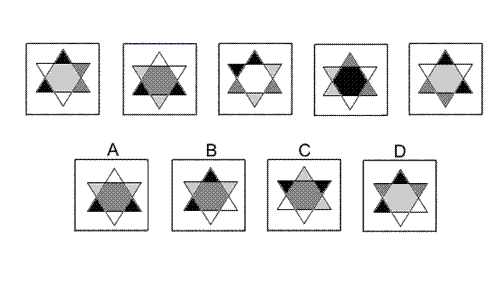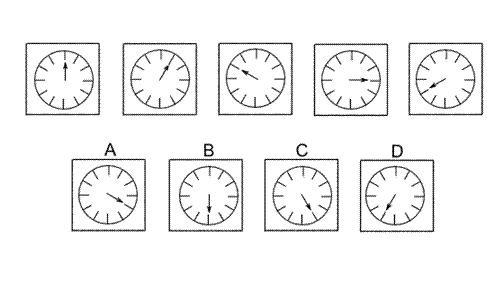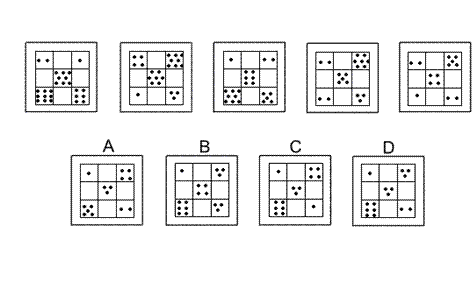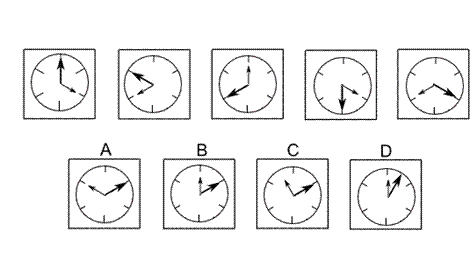Aptitude Tests 4 Me

Non Verbal Logic

Detailed solution

40.Solution: B Explanation: In this question there are regions with four colours - black, light grey, dark grey and white. There are two rules to follow at the same time. The first rule is that the star shape rotates by 60° anticlockwise about its centre each time. The second rule is that the colours of the regions change according to the pattern - black changes to light grey, light grey changes to dark grey, dark grey changes to white, and white changes to black.

41.Solution: C Explanation: In this question the arrow rotates around the clock face alternately clockwise and anticlockwise and the angle of rotation is always an odd multiple of 30°. Thus the first rotation is 30° (1 × 30°) clockwise; the next is 90° (3 × 30°) anticlockwise, the next 150° (5 × 30°) clockwise, and the next 210° (7 × 30°) anticlockwise. The final rotation to get the next diagram in the sequence will therefore be 270° (9 × 30°) clockwise, and the correct answer is C.

42.Solution: D Explanation: In this question there are three rules to follow. The first rule is that the number of dots in the centre square of the grid decreases by 1 each time. In the next diagram of the sequence, therefore, the centre square should have three dots and the correct answer could be A, C or D. The second rule is that the number of dots in the centre square is equal to the sum of the numbers of dots in the top left square and the bottom right square. The correct answer, therefore, could be A, B or D. The third rule is that the number of dots in the centre square is equal to the difference between the numbers of dots in the top right square and the bottom left square. The correct answer, therefore, can only be D.

43.a. The United States is to the world as a brick is to a brick house. This relationship shows part to whole. The United States is one part of the world; the brick is one part of the house.

 1 2 3 4 5 6 7 8 9 10 11 12 13 14 15 16 17 18 19 20 21 22 23 24 25 26 27 28 29 30 31 32 33 34 35 36 37 38 39 40 41 42 43 44 45 46 47 48 49 50 51 52 53 54 55 56 57 58 59 60 61 62 63 64 65 66 67 68 69 70 71 72 73 74 75 76 77 78 79 80 81 82 83 84 85 86 87 88Passage ReadingVerbal LogicNon Verbal LogicNumerical LogicData InterpretationReasoningAnalytical AbilityBasic NumeracyAbout UsContactPrivacy PolicyMajor TestsFAQ